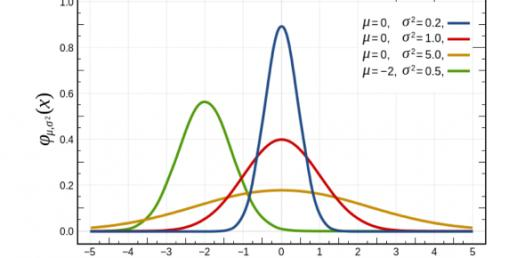# Can You Pass This AP Statistics Exam? Quiz

5 Questions | Total Attempts: 703Settings.

Related Topics
• 1.
Researchers in the Southwest studying tortoise habitat collected the following data.  If a tortoise is selected at random, let A = the tortoise is a female, and B = the tortoise is a Morafka.  Which answer appropriately interprets the value of P(B|A)? Species Male Female Agassiz 18 33 Morafka 12 15
• A.

31.3% is the probability that a randomly selected Morafka tortoise is a female tortoise.

• B.

31.3% is the probability that a randomly selected female tortoise is a Morafka tortoise.

• C.

19.2% is the probability that a randomly selected Morafka tortoise is a female tortoise.

• D.

19.2% is the probability that a randomly selected female tortoise is a Morafka tortoise.

• E.

31.3% is the probability that a randomly selected tortoise is a female tortoise.

• 2.
Which of the following is not an advantage of stratified random sampling over simple random sampling?
• A.

When done correctly, a stratified random sampling process has less variability from sample to sample than a simple random sample.

• B.

When done correctly, a stratified random sample can provide, with a smaller sample size, an estimate that is just as reliable as that of a simple random sample with a larger sample size.

• C.

A stratified random sample provides information about each stratum in the population as well as an estimate for the population as a whole, and a simple random sample does not.

• D.

When done correctly, a stratified random sample is less biased than a simple random sample.

• E.

When done correctly, a stratified random sample can provide a more reliable estimate than a simple random sample using the same sample size.

• 3.
The correlation between height in inches and weight in pounds for a particular class is 0.65.  If the heights are converted from inches to centimeters, what will the correlation be? (1 in.  = 2.54 cm)
• A.

-0.65

• B.

-0.26

• C.

0.10

• D.

0.26

• E.

0.65

• 4.
Researchers wish to test how rangelands growth is affected by wildfire.  A very large open area is divided into 28 equally sized plots and subjected to a controlled burn.  One year later, the researchers calculate the percentage of grass returned in each plot after the fire damage.  The average percentage return for these 28 plots was 58.5% with a standard deviation of 6.5%.  Which of the following represents a 95% confidence interval to estimate the average proportion of grass return one year after fire?
• A.

A

• B.

B

• C.

C

• D.

D

• E.

E

• 5.
A spreadsheet contains a data set that consists of 100 values such that IQR > variance.  However, these values must be converted using the following formula:  NewValue = 2(OldValue)+10.  Which of the following statements is true about the data set consisting of the NewValues?
• A.

The IQR has been increased by 10 units.

• B.

The IQR is now less than the variance.

• C.

The IQR is now double its previous size.

• D.

The IQR has been doubled and then increased by 10 units.

• E.

The IQR has been cut in half.